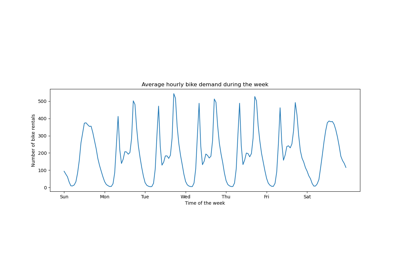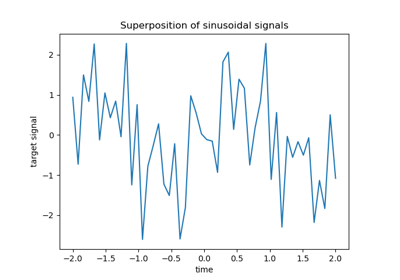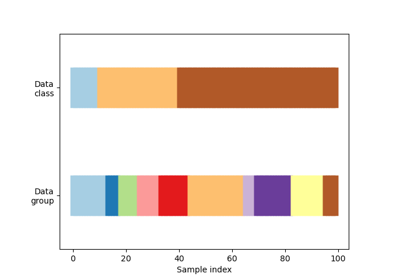# sklearn.model_selection.TimeSeriesSplit¶

class sklearn.model_selection.TimeSeriesSplit(n_splits=5, *, max_train_size=None, test_size=None, gap=0)[source]

Time Series cross-validator

Provides train/test indices to split time series data samples that are observed at fixed time intervals, in train/test sets. In each split, test indices must be higher than before, and thus shuffling in cross validator is inappropriate.

This cross-validation object is a variation of KFold. In the kth split, it returns first k folds as train set and the (k+1)th fold as test set.

Note that unlike standard cross-validation methods, successive training sets are supersets of those that come before them.

Read more in the User Guide.

For visualisation of cross-validation behaviour and comparison between common scikit-learn split methods refer to Visualizing cross-validation behavior in scikit-learn

New in version 0.18.

Parameters:
n_splitsint, default=5

Number of splits. Must be at least 2.

Changed in version 0.22: n_splits default value changed from 3 to 5.

max_train_sizeint, default=None

Maximum size for a single training set.

test_sizeint, default=None

Used to limit the size of the test set. Defaults to n_samples // (n_splits + 1), which is the maximum allowed value with gap=0.

New in version 0.24.

gapint, default=0

Number of samples to exclude from the end of each train set before the test set.

New in version 0.24.

Notes

The training set has size i * n_samples // (n_splits + 1) + n_samples % (n_splits + 1) in the i th split, with a test set of size n_samples//(n_splits + 1) by default, where n_samples is the number of samples.

Examples

>>> import numpy as np
>>> from sklearn.model_selection import TimeSeriesSplit
>>> X = np.array([[1, 2], [3, 4], [1, 2], [3, 4], [1, 2], [3, 4]])
>>> y = np.array([1, 2, 3, 4, 5, 6])
>>> tscv = TimeSeriesSplit()
>>> print(tscv)
TimeSeriesSplit(gap=0, max_train_size=None, n_splits=5, test_size=None)
>>> for i, (train_index, test_index) in enumerate(tscv.split(X)):
...     print(f"Fold {i}:")
...     print(f"  Train: index={train_index}")
...     print(f"  Test:  index={test_index}")
Fold 0:
Train: index=
Test:  index=
Fold 1:
Train: index=[0 1]
Test:  index=
Fold 2:
Train: index=[0 1 2]
Test:  index=
Fold 3:
Train: index=[0 1 2 3]
Test:  index=
Fold 4:
Train: index=[0 1 2 3 4]
Test:  index=
>>> # Fix test_size to 2 with 12 samples
>>> X = np.random.randn(12, 2)
>>> y = np.random.randint(0, 2, 12)
>>> tscv = TimeSeriesSplit(n_splits=3, test_size=2)
>>> for i, (train_index, test_index) in enumerate(tscv.split(X)):
...     print(f"Fold {i}:")
...     print(f"  Train: index={train_index}")
...     print(f"  Test:  index={test_index}")
Fold 0:
Train: index=[0 1 2 3 4 5]
Test:  index=[6 7]
Fold 1:
Train: index=[0 1 2 3 4 5 6 7]
Test:  index=[8 9]
Fold 2:
Train: index=[0 1 2 3 4 5 6 7 8 9]
Test:  index=[10 11]
>>> # Add in a 2 period gap
>>> tscv = TimeSeriesSplit(n_splits=3, test_size=2, gap=2)
>>> for i, (train_index, test_index) in enumerate(tscv.split(X)):
...     print(f"Fold {i}:")
...     print(f"  Train: index={train_index}")
...     print(f"  Test:  index={test_index}")
Fold 0:
Train: index=[0 1 2 3]
Test:  index=[6 7]
Fold 1:
Train: index=[0 1 2 3 4 5]
Test:  index=[8 9]
Fold 2:
Train: index=[0 1 2 3 4 5 6 7]
Test:  index=[10 11]


Methods

 Get metadata routing of this object. get_n_splits([X, y, groups]) Returns the number of splitting iterations in the cross-validator split(X[, y, groups]) Generate indices to split data into training and test set.

Get metadata routing of this object.

Please check User Guide on how the routing mechanism works.

Returns:

A MetadataRequest encapsulating routing information.

get_n_splits(X=None, y=None, groups=None)[source]

Returns the number of splitting iterations in the cross-validator

Parameters:
Xobject

Always ignored, exists for compatibility.

yobject

Always ignored, exists for compatibility.

groupsobject

Always ignored, exists for compatibility.

Returns:
n_splitsint

Returns the number of splitting iterations in the cross-validator.

split(X, y=None, groups=None)[source]

Generate indices to split data into training and test set.

Parameters:
Xarray-like of shape (n_samples, n_features)

Training data, where n_samples is the number of samples and n_features is the number of features.

yarray-like of shape (n_samples,)

Always ignored, exists for compatibility.

groupsarray-like of shape (n_samples,)

Always ignored, exists for compatibility.

Yields:
trainndarray

The training set indices for that split.

testndarray

The testing set indices for that split.

## Examples using sklearn.model_selection.TimeSeriesSplit¶Time-related feature engineering

Time-related feature engineeringL1-based models for Sparse Signals

L1-based models for Sparse SignalsVisualizing cross-validation behavior in scikit-learn

Visualizing cross-validation behavior in scikit-learn